Latest Banking jobs   »

# Quantitative Aptitude Quiz For SBI Clerk Prelims 2022- 23rd May

Directions (1–5): In each of these questions, two equations (I) and (II) are given. You have to solve both the equations and answer the following questions.
(a) x>y
(b) x≥y
(c) x=y or no relation.
(d) x<y
(e) x≤y

Q1. I. 28x² – 41x + 15 = 0
II. 35y² – 43y + 12 = 0

Q2. I. 2x² – 26x + 80 = 0
II. 2y² – 38y + 176 = 0

Q3. I. 7x – 9y + 51 = 0
II. 13y – 11x – 63 = 0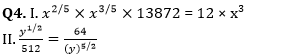Q5. I. x² + 25x + 156 = 0
II. y² + 21y + 110 = 0

Directions (6-10): In each question two equations numbered (I) and (II) are given. Student should solve both the equations and mark appropriate answer.
(a) If x=y or no relation can be established
(b) If x>y
(c) If x<y
(d) If x≥y
(e) If x≤y

Q6. I . x² + 13x + 42 = 0
II. y² + 12y + 36 = 0

Q7. I. x² + 2x – 143 = 0
II. 2y² + 4y – 286 = 0

Q8. I. x² = 1369
II. y³ + 57373 = 6720

Q9. I. 19x + 17y = 220
II. 17x + 19y = 212

Q10. I. 2x² + 20x = –50
II. y² = 25

Directions (11–15): In each of these questions, two equations (I) and (II) are given. You have to solve both the equations and answer the following questions.
(a) x < y
(b) x ≥ y
(c) x > y
(d) x ≤ y
(e) x = y or no relation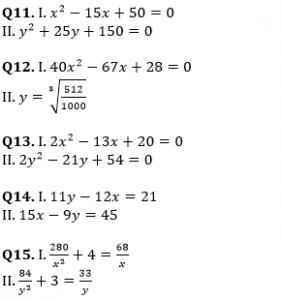Solutions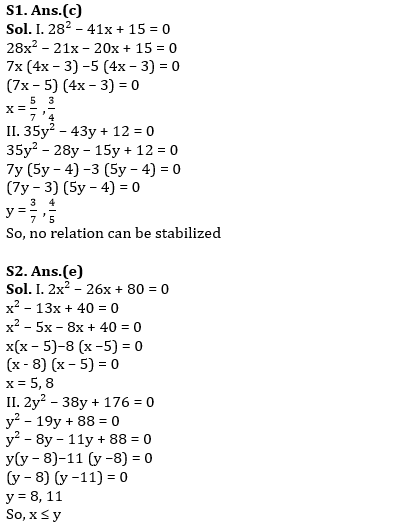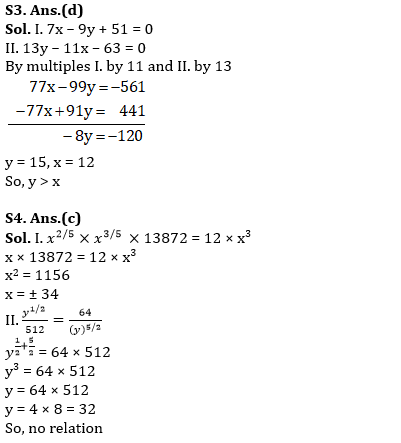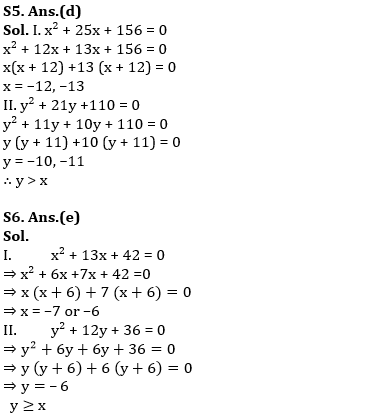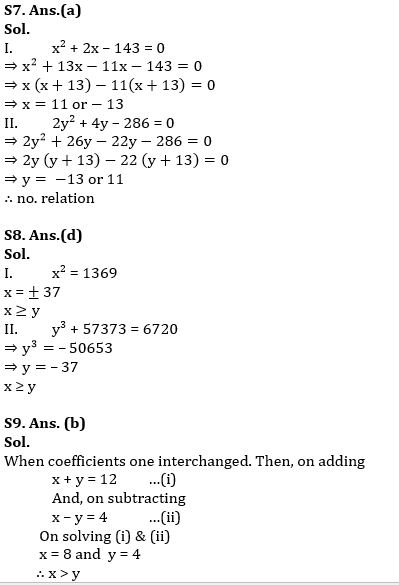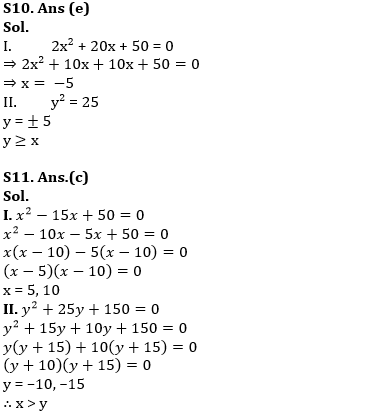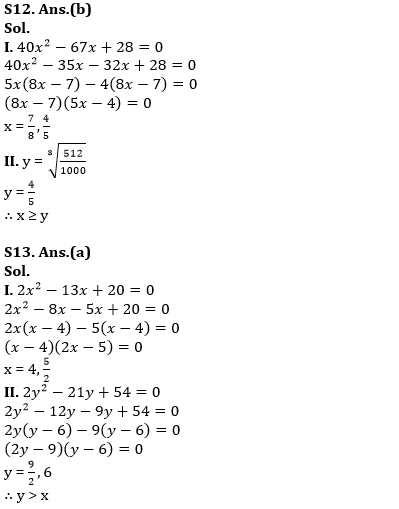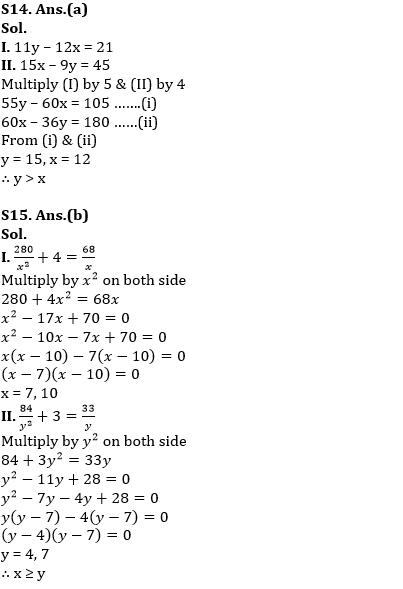#### Congratulations!